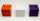# Cube + area - math problems

#### Number of problems found: 110

• CubeThe sum of all cube edges is 30cm. Find the surface area of the cube.
• Cube surface and volumeFind the surface of the cube with a volume of 27 dm3.
• Minimum surfaceFind the length, breadth, and height of the cuboid-shaped box with a minimum surface area, into which 50 cuboid-shaped blocks, each with length, breadth, and height equal to 4 cm, 3 cm, and 2 cm respectively, can be packed.
• Cube wallsFind the volume and the surface area of the cube if the area of one of its walls is 40 cm2.
• Cube edgesThe sum of the lengths of the cube edges is 42 cm. Calculate the surface of the cube.
• Big cubeCalculate the surface of the cube, which is composed of 64 small cubes with an edge 1 cm long.
• Surface of cubesPeter molded a cuboid 2 cm, 4cm, 9cm of plasticine. Then the plasticine split into two parts in a ratio 1:8. From each piece made a cube. In what ratio are the surfaces of these cubes?
• Three cubesThe body was created by gluing three identical cubes. Its volume is 192 cm3. What is its surface in dm2?
• Sheet of paperIs the sheet of paper measuring 18 and 25 cm enough for taping 9 cm cubes with an edge? (1 = yes, 0 = no)
• Cube surface areaThe surface of the cube was originally 216 centimeters square. The surface of the cube has shrunk from 216 to 54 centimeters sq. Calculate how much percent the edge of the cube has decreased.
• Cube 1-2-3Calculate the volume and surface area of the cube ABCDEFGH if: a) /AB/ = 4 cm b) perimeter of wall ABCD is 22 cm c) the sum of the lengths of all edges of the cube is 30 cm.
• CubeOne cube has an edge increased five times. How many times will larger it's surface area and volume?
• Surface of wallFind by what percentage the surface of the cube will decrease if we reduce the surface of each of its walls by 12%.
• Area of a cubeCalculate the surface area of a cube if its volume is equal to 729 cubic meters.
• Cube edgeDetermine the edges of the cube when the surface is equal to 37.5 cm square.
• Volume and areaWhat is the volume of a cube which has an area of 361 cm2?
• Prism basesVolume perpendicular quadrilateral prism is 360 cm3. The edges of the base and height of the prism are in the ratio 5:4:2. Determine the area of the base and walls of the prism.
• The cube - simpleCalculate the surface of the cube measuring 15 centimeters.
• The cubeThe cube has a surface area of 486 m ^ 2. Calculate its volume.
• Cube surface areaThe Wall of the cube has a content area 99 cm square. What is the surface of the cube?

Do you have an exciting math question or word problem that you can't solve? Ask a question or post a math problem, and we can try to solve it.

We will send a solution to your e-mail address. Solved examples are also published here. Please enter the e-mail correctly and check whether you don't have a full mailbox.

Cube Problems. Area - math problems.